GeeksforGeeks App
Open AppBrowser
Continue

# Transportation Problem | Set 4 (Vogel’s Approximation Method)

The North-West Corner method and the Least Cost Cell method has been discussed in the previous articles. In this article, the Vogel’s Approximation method will be discussed.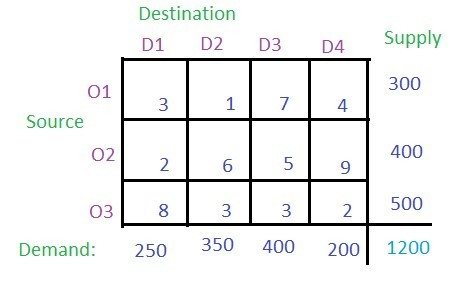Solution:

• For each row find the least value and then the second least value and take the absolute difference of these two least values and write it in the corresponding row difference as shown in the image below. In row O1, 1 is the least value and 3 is the second least value and their absolute difference is 2. Similarly, for row O2 and O3, the absolute differences are 3 and 1 respectively.
• For each column find the least value and then the second least value and take the absolute difference of these two least values then write it in the corresponding column difference as shown in the figure. In column D1, 2 is the least value and 3 is the second least value and their absolute difference is 1. Similarly, for column D2, D3 and D3, the absolute differences are 2, 2 and 2 respectively.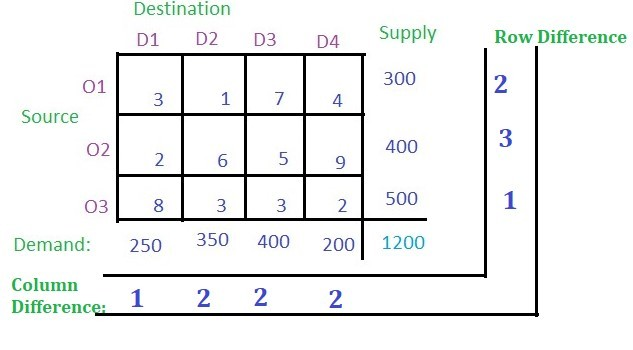• These value of row difference and column difference are also called as penalty. Now select the maximum penalty. The maximum penalty is 3 i.e. row O2. Now find the cell with the least cost in row O2 and allocate the minimum among the supply of the respective row and the demand of the respective column. Demand is smaller than the supply so allocate the column’s demand i.e. 250 to the cell. Then cancel the column D1.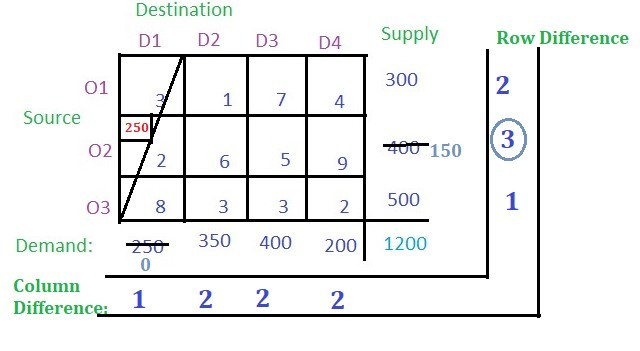• From the remaining cells, find out the row difference and column difference.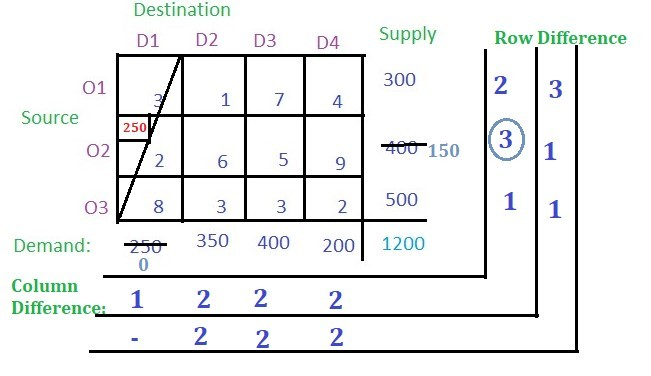• Again select the maximum penalty which is 3 corresponding to row O1. The least-cost cell in row O1 is (O1, D2) with cost 1. Allocate the minimum among supply and demand from the respective row and column to the cell. Cancel the row or column with zero value.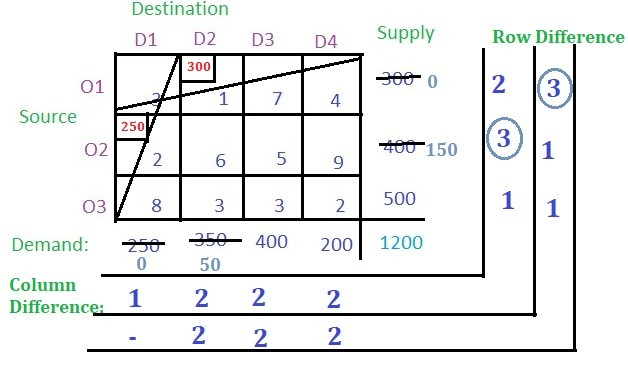• Now find the row difference and column difference from the remaining cells.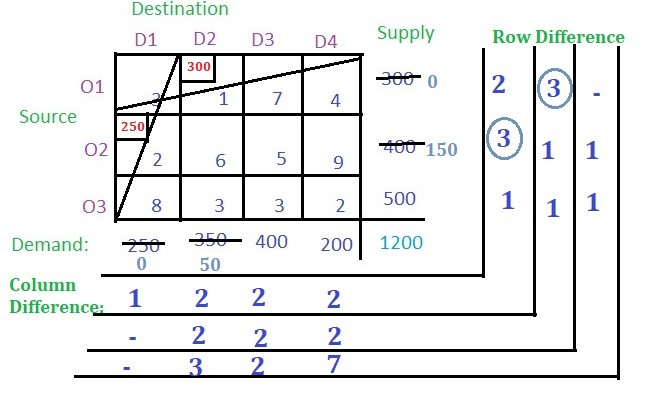• Now select the maximum penalty which is 7 corresponding to column D4. The least cost cell in column D4 is (O3, D4) with cost 2. The demand is smaller than the supply for cell (O3, D4). Allocate 200 to the cell and cancel the column.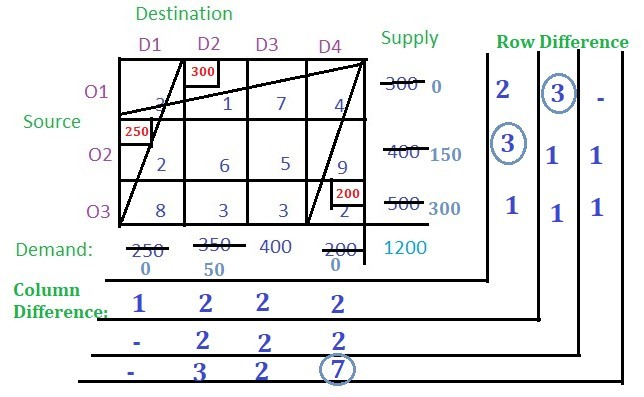• Find the row difference and the column difference from the remaining cells.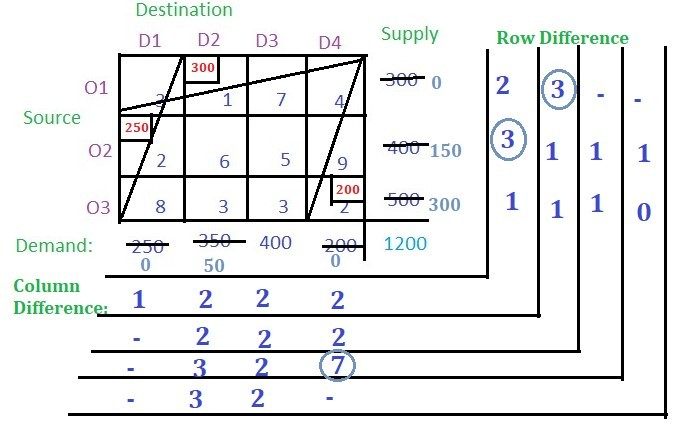• Now the maximum penalty is 3 corresponding to the column D2. The cell with the least value in D2 is (O3, D2). Allocate the minimum of supply and demand and cancel the column.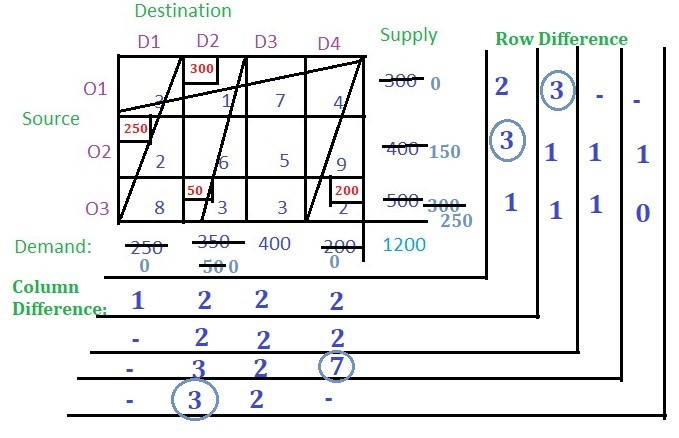• Now there is only one column so select the cell with the least cost and allocate the value.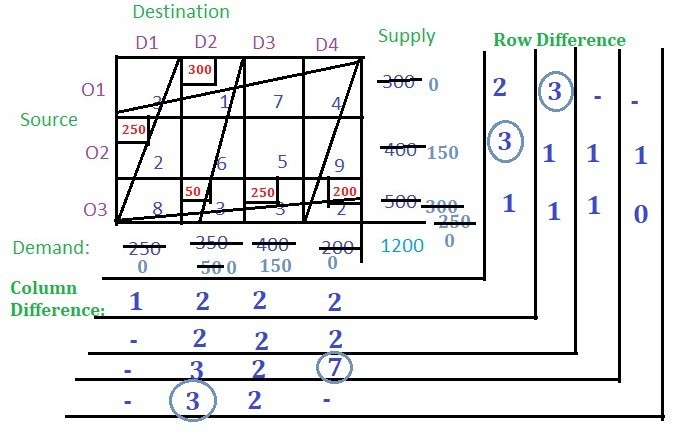• Now there is only one cell so allocate the remaining demand or supply to the cell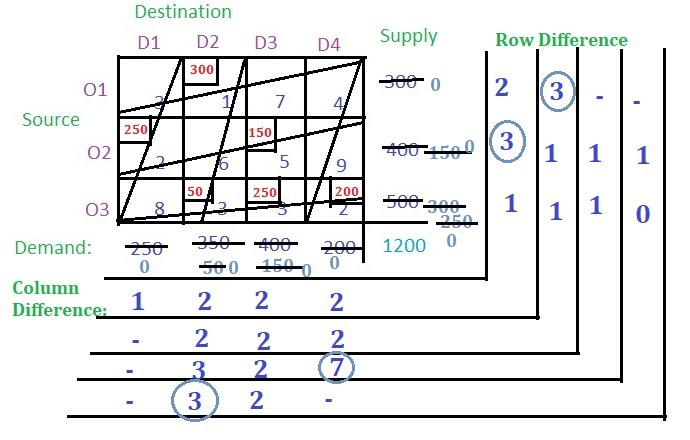• No balance remains. So multiply the allocated value of the cells with their corresponding cell cost and add all to get the final cost i.e. (300 * 1) + (250 * 2) + (50 * 3) + (250 * 3) + (200 * 2) + (150 * 5) = 2850

Below is the implementation for the approach discussed above

## Python3

 `grid ``=` `[[``3``, ``1``, ``7``, ``4``], [``2``, ``6``, ``5``, ``9``], [``8``, ``3``, ``3``, ``2``]]  ``# table``supply ``=` `[``300``, ``400``, ``500``]  ``# supply``demand ``=` `[``250``, ``350``, ``400``, ``200``]  ``# demand``INF ``=` `10``*``*``3``n ``=` `len``(grid)``m ``=` `len``(grid[``0``])``ans ``=` `0` `# hepler function for finding the row difference and the column difference``def` `findDiff(grid):``    ``rowDiff ``=` `[]``    ``colDiff ``=` `[]``    ``for` `i ``in` `range``(``len``(grid)):``        ``arr ``=` `grid[i][:]``        ``arr.sort()``        ``rowDiff.append(arr[``1``]``-``arr[``0``])``    ``col ``=` `0``    ``while` `col < ``len``(grid[``0``]):``        ``arr ``=` `[]``        ``for` `i ``in` `range``(``len``(grid)):``            ``arr.append(grid[i][col])``        ``arr.sort()``        ``col ``+``=` `1``        ``colDiff.append(arr[``1``]``-``arr[``0``])``    ``return` `rowDiff, colDiff`  `# loop runs until both the demand and the supply is exhausted``while` `max``(supply) !``=` `0` `or` `max``(demand) !``=` `0``:``    ``# finding the row and col difference``    ``row, col ``=` `findDiff(grid)``    ``# finding the maxiumum element in row difference array``    ``maxi1 ``=` `max``(row)``    ``# finding the maxiumum element in col difference array``    ``maxi2 ``=` `max``(col)` `    ``# if the row diff max element is greater than or equal to col diff max element``    ``if``(maxi1 >``=` `maxi2):``        ``for` `ind, val ``in` `enumerate``(row):``            ``if``(val ``=``=` `maxi1):``                ``# finding the minimum element in grid index where the maximum was found in the row difference``                ``mini1 ``=` `min``(grid[ind])``                ``for` `ind2, val2 ``in` `enumerate``(grid[ind]):``                    ``if``(val2 ``=``=` `mini1):``                        ``# calculating the min of supply and demand in that row and col``                        ``mini2 ``=` `min``(supply[ind], demand[ind2])``                        ``ans ``+``=` `mini2 ``*` `mini1``                        ``# subtracting the min from the supply and demand``                        ``supply[ind] ``-``=` `mini2``                        ``demand[ind2] ``-``=` `mini2``                        ``# if demand is smaller then the entire col is assigned max value so that the col is eliminated for the next iteration``                        ``if``(demand[ind2] ``=``=` `0``):``                            ``for` `r ``in` `range``(n):``                                ``grid[r][ind2] ``=` `INF``                        ``# if supply is smaller then the entire row is assigned max value so that the row is eliminated for the next iteration``                        ``else``:``                            ``grid[ind] ``=` `[INF ``for` `i ``in` `range``(m)]``                        ``break``                ``break``    ``# if the row diff max element is greater than col diff max element``    ``else``:``        ``for` `ind, val ``in` `enumerate``(col):``            ``if``(val ``=``=` `maxi2):``                ``# finding the minimum element in grid index where the maximum was found in the col difference``                ``mini1 ``=` `INF``                ``for` `j ``in` `range``(n):``                    ``mini1 ``=` `min``(mini1, grid[j][ind])` `                ``for` `ind2 ``in` `range``(n):``                    ``val2 ``=` `grid[ind2][ind]``                    ``if` `val2 ``=``=` `mini1:``                        ``# calculating the min of supply and demand in that row and col``                        ``mini2 ``=` `min``(supply[ind2], demand[ind])``                        ``ans ``+``=` `mini2 ``*` `mini1``                        ``# subtracting the min from the supply and demand``                        ``supply[ind2] ``-``=` `mini2``                        ``demand[ind] ``-``=` `mini2``                        ``# if demand is smaller then the entire col is assigned max value so that the col is eliminated for the next iteration``                        ``if``(demand[ind] ``=``=` `0``):``                            ``for` `r ``in` `range``(n):``                                ``grid[r][ind] ``=` `INF``                        ``# if supply is smaller then the entire row is assigned max value so that the row is eliminated for the next iteration``                        ``else``:``                            ``grid[ind2] ``=` `[INF ``for` `i ``in` `range``(m)]``                        ``break``                ``break` `print``(``"The basic feasible solution is "``, ans)`

My Personal Notes arrow_drop_up